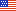An unofficial archive of your favorite United States government website

# Computer Security Resource Center

A  |  B  |  C  |  D  |  E  |  F  |  G  |  H  |  I  |  J  |  K  |  L  |  M  |  N  |  O  |  P  |  Q  |  R  |  S  |  T  |  U  |  V  |  W  |  X  |  Y  |  Z

### min-entropy

Abbreviation(s) and Synonym(s):

None

Definition(s):

The min-entropy (in bits) of a random variable X is the largest value m having the property that each observation of X provides at least m bits of information (i.e., the min-entropy of X is the greatest lower bound for the information content of potential observations of X). The min-entropy of a random variable is a lower bound on its entropy. The precise formulation for min-entropy is −(log2 max pi) for a discrete distribution having n possible outputs with probabilities p1,…, pn. Min-entropy is often used as a worst-case measure of the unpredictability of a random variable. Also see [NIST SP 800-90B].
Source(s):
NIST SP 800-90A Rev. 1 under Min-entropy

The min-entropy (in bits) of a random variable X is the largest value m having the property that each observation of X provides at least m bits of information (i.e., the min-entropy of X is the greatest lower bound for the information content of potential observations of X). The min-entropy of a random variable is a lower bound on its entropy. The precise formulation for min-entropy is (log2 max pi) for a discrete distribution having probabilities p1, ...,pk. Min-entropy is often used as a worst-case measure of the unpredictability of a random variable.
Source(s):
NIST SP 800-90B under Min-entropy

A measure of the difficulty that an Attacker has to guess the most commonly chosen password used in a system. In this document, entropy is stated in bits. When a password has n-bits of min-entropy then an Attacker requires as many trials to find a user with that password as is needed to guess an n-bit random quantity. The Attacker is assumed to know the most commonly used password(s). See Appendix A.
Source(s):
NIST SP 800-63-2 under Min-entropy [Superseded]